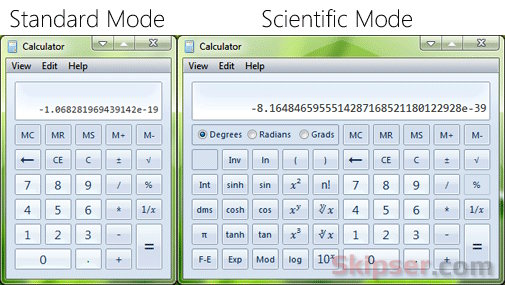The calculator, better known as the "calc" has been an integral component of Windows since 1989 when windows version 3.0 was introduced. A lot has changed in Windows over the years, but apart from the look and feel, the good old calculator has remained almost the same.

Now can you imagine a bug that existed in the calculator since it was first introduced and was never fixed by the Microsoft guys? Oh yes, there is a bug and a very stupid one indeed.

Here's how you can see it - Just try to subtract 2 from the square root of 4.

sqrt(4)-2

Square root of 4 is 2 and "2-2" should give 0. But here's the surprise - our calculator would give a small negative number as the result instead of 0. In "Standard" mode, you would see the result "-1.068281969439142e-19 " and in the "Scientific" mode, the result would be "-8.1648465955514287168521180122928e-39".In fact, you can reproduce the error with any similar calculation like "sqrt(9)-3" or "sqrt(16)-4" and so on. Here's a video of the bug in action -

The real reason for the bug is the way calculator handles sqrt operations. The results are stored as floating point numbers instead of integers and the small precision error when it comes to floating point calculations is what you see.

It is definitely a bug which either the super intelligent Microsoft techies never found or simply didn't bother to fix. Never the less, I am sure you will ask yourself "Should I double check" the next time you use the calculator :)